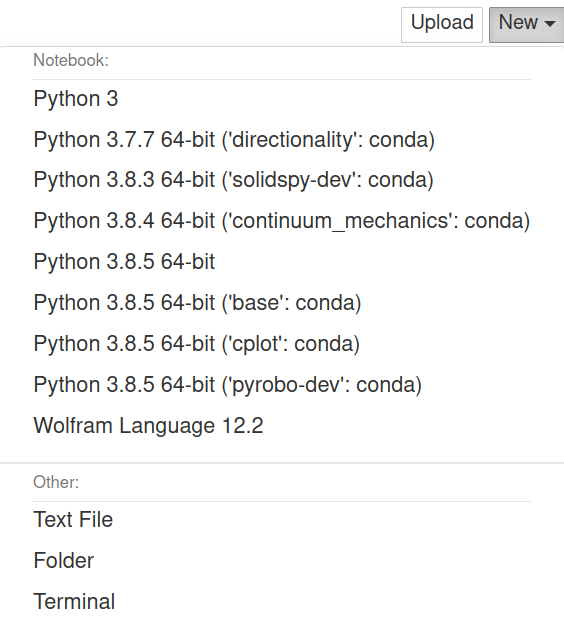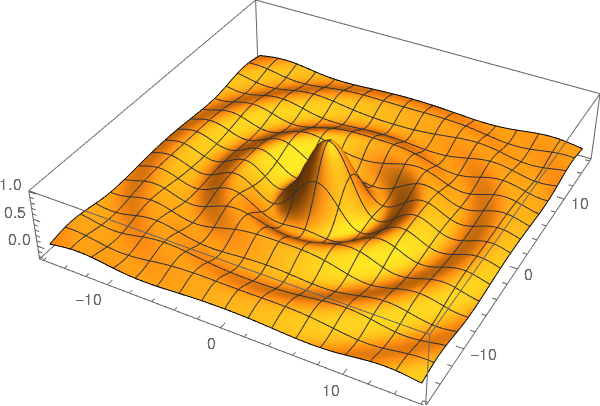# Using Wolfram Language in Jupyter: A free alternative to Mathematica

In this post I am going to describe how to add the Wolfram Language to the Jupyter notebook. This provides a free alternative to Mathematica with, pretty much, the same syntax. The use of the Wolfram Engine is free for non-production as described in their website:

The Free Wolfram Engine for Developers is available for non-production software development.

You can use this product to:

• develop a product for yourself or your company

• conduct personal projects at home, at school, at work

• explore the Wolfram Language for future production projects

## Installation

To install you should do the following steps:

• Create a Wolfram account, if you don't have one.

• Execute the installer.

• Type the following in a terminal

wolframscript


After that you should be in a terminal and see the following

Wolfram Engine activated. See https://www.wolfram.com/wolframscript/ for more information.
Wolfram Language 12.2.0 Engine for Linux x86 (64-bit)


And we can try that it is working

In:= \$Version

Out= 12.2.0 for Linux x86 (64-bit) (January 7, 2021)

In:= Integrate[1/(1 + x^2), x]

Out= ArcTan[x]


Now we need to install WolframLanguageForJupyter. For that we can type the following in a terminal

git clone https://github.com/WolframResearch/WolframLanguageForJupyter.git

cd WolframLanguageForJupyter/



To test that it is installed we can type the following in a terminal

jupyter kernelspec list


and it should have an output that includes a line similar to the following

wolframlanguage12.   /home/nicoguaro/.local/share/jupyter/kernels/wolframlanguage12.2


Or we could also try with

jupyter notebook


and see the following in the kernel menu.## Test drive

I tested some of the capabilities and you can download the notebook or see a static version here.

Let's compute the integral

\begin{equation*} \int \frac{1}{1 + x^3}\mathrm{d}x\, . \end{equation*}
sol:= Integrate[1/(1 + x^3), x]
TeXForm[sol]

\begin{equation*} -\frac{1}{6} \log \left(x^2-x+1\right)+\frac{1}{3} \log (x+1)+\frac{\tan^{-1}\left(\frac{2 x-1}{\sqrt{3}}\right)}{\sqrt{3}} \end{equation*}

And make a 3D plot.

fun:= Sin[Sqrt[x^2 + y^2]]/Sqrt[x^2 + y^2]
Plot3D[fun, {x, -5*Pi, 5*Pi}, {y, -5*Pi, 5*Pi},
PlotPoints -> 100, BoxRatios -> {1, 1, 0.2},
PlotRange -> All]In this case we don't have an interactive image. This is still not implemented, but if you are interested there is an open issue about it in GitHub.